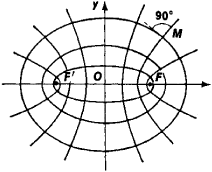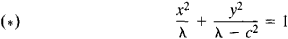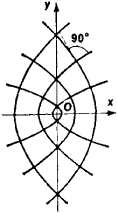# Confocal Conics

## confocal conics

[kän′fō·kəl ′kän·iks]
(mathematics)
A system of ellipses and hyperbolas that have the same pair of foci.
A system of parabolas that have the same focus and the same axis of symmetry.
McGraw-Hill Dictionary of Scientific & Technical Terms, 6E, Copyright © 2003 by The McGraw-Hill Companies, Inc.
The following article is from The Great Soviet Encyclopedia (1979). It might be outdated or ideologically biased.

## Confocal Conics

quadric curves with common foci. If F and F are two given points in the plane, then through each point of the plane there pass a single ellipse and a single hyperbola having F and F as their foci (Figure 1). Every ellipse is orthogonal to any hyperbola with which it is confocal—that is, the ellipse and the hyperbola intersect (at four points) at right angles (the angle between two intersecting curves is defined as the angle between the tangents to the curves at the point of intersection).Figure 1

The set of all conformal ellipses and hyperbolas in an appropriate coordinate system is defined by the equationwhere c is the distance of the foci from the origin and λ is a variable parameter. When λ > c2, the equation defines an ellipse. When 0 < λ < c2, it defines a hyperbola, and when λ < 0, an imaginary conic. If one of the foci tends to infinity, two families of confocal parabolas are obtained at the limit (Figure 2). Any two parabolas in different families are orthogonal.Figure 2

An elliptic coordinate system can be introduced into the plane by means of confocal ellipses and hyperbolas. Thus, if M(x, y) is an arbitrary point in the plane, a quadratic equation in λ can be obtained by substituting the coordinates of the point for x and y in equation (*). The roots λ1 and λ2 of this quadratic equation are the elliptic coordinates of M. The coordinate curves of an elliptic coordinate system are the confocal ellipses and hyperbolas defined by the equations λ1 = const and λ2 = const.